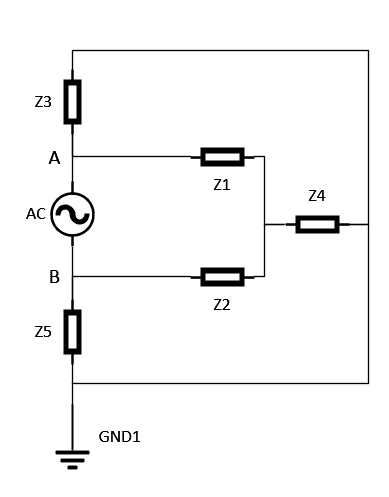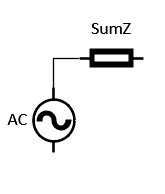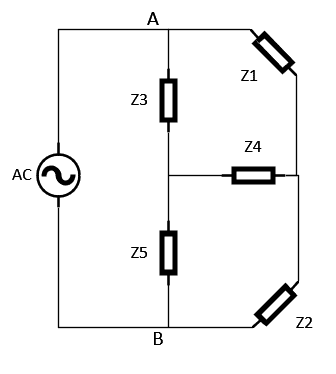# Thevenin equivalent (symbolic circuit calculation)

• Casper Hansen
You will see that the current flowing through the circuit is equal to the voltage across the resistor at node A multiplied by the sum of the impedances in series and in parallel at node B.

## Homework Statement

I have trouble to find the right procedure to transform the circuit below to a thevenin equivalent.
I am looking for a symbolic solution a step by step guidance of where to start and how to add/locate all the impedance in series and parallel in order to calculate the sum of Z seen from point A and B## The Attempt at a Solution

[/B]
I have a hard time see which impedance are in series and parallel, as Z5, Z4 and Z3 forms a star connection to GND?Would the solution be to make a form of star/delta transformation of the circuit in order to calculate the circuit?

#### Attachments

Last edited:
You have to define the nodes between which you want the Thevenin equivalent. Without that your question has no answer.

Also, we don't DO "step by step" instructions. YOU do a step by step analysis and tell us when you get stuck.

phinds said:
You have to define the nodes between which you want the Thevenin equivalent. Without that your question has no answer.

Also, we don't DO "step by step" instructions. YOU do a step by step analysis and tell us when you get stuck.

My post have been updated with nodes A and B

Casper Hansen said:
My post have been updated with nodes A and B
Then a first step of getting the Thevenin Equivalent voltage should be REALLY easy. What is it?

phinds said:
Then a first step of getting the Thevenin Equivalent voltage should be REALLY easy. What is it?
If i know the voltage but need to find the sum of impedances in order to calculate the current

Casper Hansen said:
If i know the voltage but need to find the sum of impedances in order to calculate the current
You did not answer my question.

Casper Hansen said:
If i know the voltage but need to find the sum of impedances in order to calculate the current
You can find the current by using standard circuit analysis methods: basic KVL & KCL, Nodal Analysis, Mesh analysis... Take your pick.

Edit: Or, as you've stated, you could use a star-delta transform to begin reduction of the resistor network.

phinds said:
You did not answer my question.
Sorry I did not quite get what you are asking for?

Casper Hansen said:
Sorry I did not quite get what you are asking for?
"Then a first step of getting the Thevenin Equivalent voltage should be REALLY easy. What is it?" is asking you for the Thevenin Equivalent voltage. I don't see how I could have asked it any more plainly. What is the Thevenin Equivalent voltage, given the circuit you have drawn with the nodes you have drawn? Just LOOK.